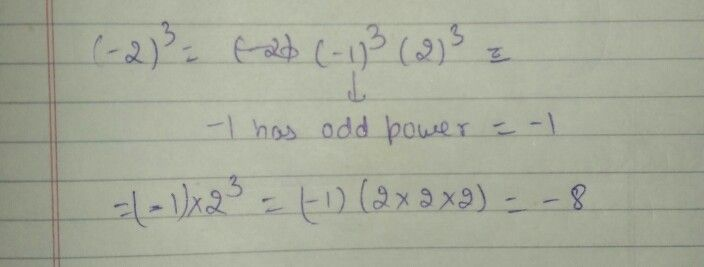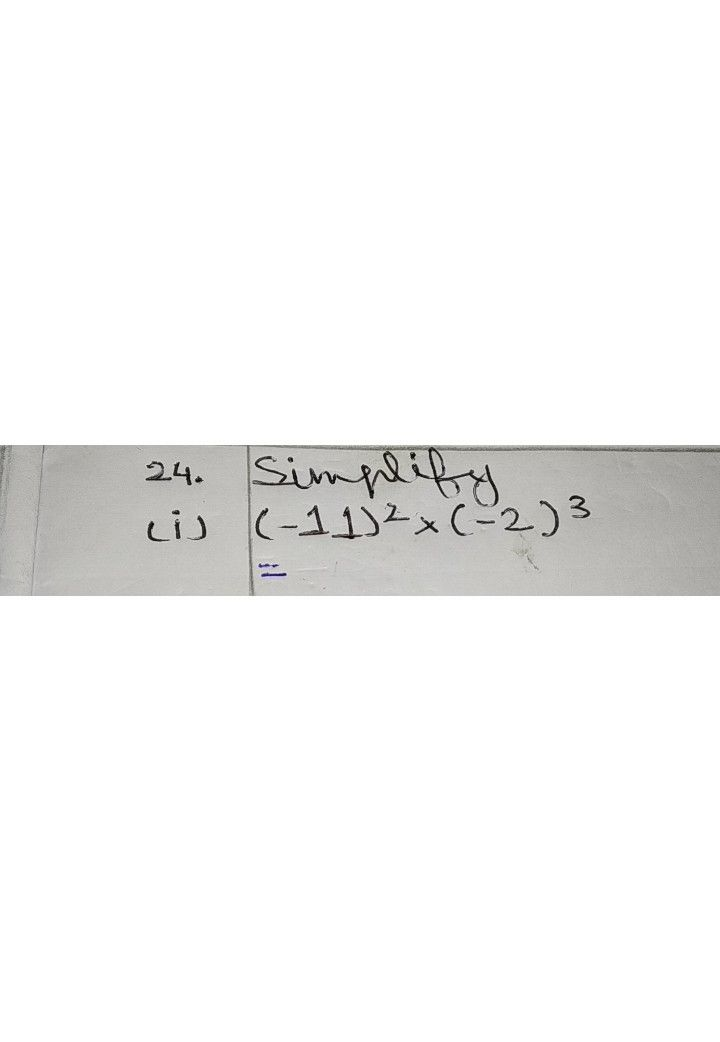Symbol
Problem$22$ $°$ $Ci\right)$ Find the ealue $°$ $c1^{1}\right)$ $\left(-2$ $\right)3$ $23$ $2^{3}=522actim23$ $2$ $2^{3}=2\times 2\times 2=4\times 2$ times $=8$ $-$ $1$ $-$ $-$
7th-9th grade
Other
SolutionQanda teacher - Akanksha09sorry i was solving another question so i could not see Your msg earlier
kindly wait for some time for rply as sometimes i am doong another questionsStudent
ok mamQanda teacher - Akanksha09
yes please rate my answerStudent
please tell me mam
its urgent
can i ask you a doubt mamQanda teacher - Akanksha09
yesStudentma i sent yoy
mam i sent to you
reply mam
please
please mam
mam
i delete this app right niw
please mam replyQanda teacher - Akanksha09
hello
(-11)×(-11)=121
(-2)×(-2)×(-2)=-8
121×(-8)= -968